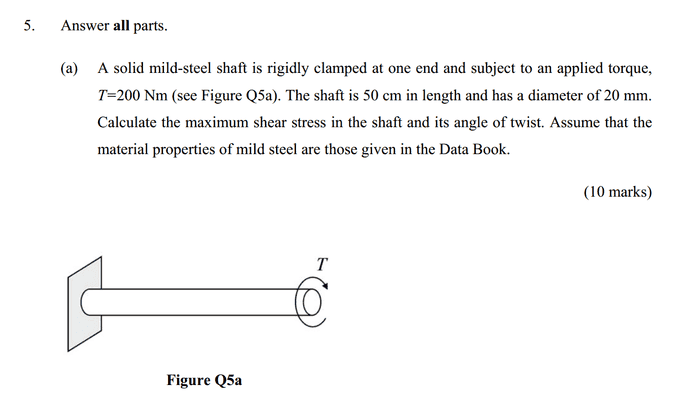# Calculating the maximum shear stress in a shaft

influx

## Homework Statement## Homework Equations

Torque = Force x Distance
Shear stress = Force/Area

3. The Attempt at a Solution
I know how to do this question as I've seen the mark scheme. However, initially I did it wrong. What I did was as follows:

Torque = Force x Distance
therefore Torque/Distance = Force
therefore Force = 200/(50/100) = 400 N

Then I used Shear stress = Force/Area and plugged in the value of 400N and that of the area to find the shear stress.

As I said, I know that this is not the way to calculate the answer to this question.
However, I want to know, what shear stress does the shear stress obtained via this method actually refer to? As in, when I did the force (that I found via T=Fr) divided by the area of the shaft, I got a value for shear stress, what is this value referring to? The shear stress at the end of the shaft? Or?